Using Interval Histograms to Make Initial Cardinality Estimates | Vantage - 17.10 - Using Interval Histograms to Make Initial Cardinality Estimates - Advanced SQL Engine - Teradata Database

Teradata Vantage™ - SQL Request and Transaction Processing

Product
Release Number
17.10
Release Date
July 2021
Content Type
Programming Reference
User Guide
Publication ID
B035-1142-171K
Language
English (United States)

The following set of examples uses a small subset of the interval histograms for the column or index values evaluated. The examples are oversimplified to emphasize basic aspects of the process.

To simplify the examples., the data demographics of the tables examined in these examples are such that their data is stored in equal-height intervals.

Note that what is described here is only the initial estimation of cardinalities, which is based on the statistics stored in the interval histograms. The Optimizer adjusts its cardinality estimates dynamically during the course of query optimization based on information derived from various database constraints, query predicates, and hash and join indexes. Because of the way the system calculates these statistics, they are referred to as derived statistics (see Derived Statistics for details).

The example sets that follow do not deal with derived statistics (see Derived Statistics). They are meant to indicate only how the Optimizer would makes its cardinality estimates if it only had the statistics in the interval histograms as a basis for making those estimates.

The Optimizer also uses UDI count data from DBC.ObjectUsage to estimate cardinalities. See Object Use and UDI Counts for further information.

Interval Histogram Data

Recall that the principal information stored in a standard equal-height interval is as follows:
• Maximum value in the interval.
• Most frequent value in the interval.

Recorded as the modal value for the interval.

• Cardinality of the most frequent value in the interval.

Recorded as the modal frequency for the interval.

• Cardinality of distinct values in the interval as determined from a full-table scan.
• Cardinality of values in the interval not equal to the most frequent value.

This number is constant across intervals when equal-height intervals are used.

Its value is calculated as follows: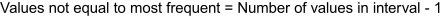where the factor 1 indicates the number of distinct values that occur most frequently in the interval.

• Cardinality of rows not containing the most frequent value in the interval.

Recorded as the non-modal frequency for the interval.

The data used for the examples is tabulated in the following table. Notice that the total number of distinct values (the shaded cells of the table) is the same for all five intervals. This is definitive, in theory, for equal-height interval histograms. In practice, the cardinalities of intervals of an equi-height interval are only approximately equal. To simplify the examples, no history intervals are shown.

Variable Interval Number
1   2   3   4   5
Instances of the most frequent value in the interval 16   36   39   60   67
Number of values not equal to the most frequent value in the interval 10   10   10   10   10
Number of distinct values in the interval 11   11   11   11   11
Number of rows in the interval having the most frequent value 50   70   20   30   50
Number of rows in the interval not having the most frequent value 200 150 250 100 200
Number of rows in the interval 250 220 270 130 250
Maximum value in the interval 25   37   50   63   76

The following diagram illustrates these numbers graphically. The hatched area represents the number of rows for other values in the interval. Some of the values have superscript explanatory notes that are defined in the table following the illustration.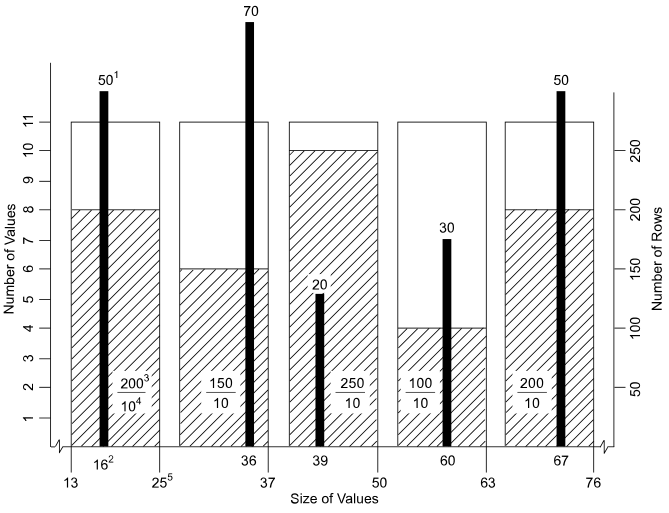Graphic Note Number Description
1 Number of rows that have the most frequent value in the interval as the value for the column or index.
2 Most frequent value for the column or index in the interval.
3 Number of rows that do not have the most frequent value in the interval as the value for the column or index.
4 Number of distinct values in the interval that are not equal to the most frequent value for the column or index.
5 Maximum value in the interval.

If the cardinality of the response set for this simple query need not be estimated because the statistics on the column set are current, then the Optimizer knows exactly what the cardinality is. There are 30 instances of the value 60 in table. This value is known because it is the count of the number of rows in the interval having the most frequent value, 60, for the column named in condition.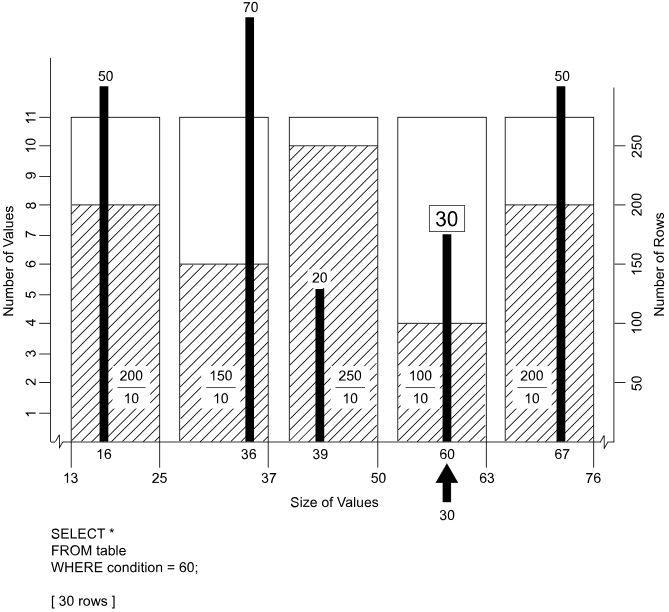This example illustrates a simple equality condition.

If there are any rows that satisfy the condition where the value for the named column is 55, they are found in the range between 51, the lower bound for the interval, and its upper bound of 63.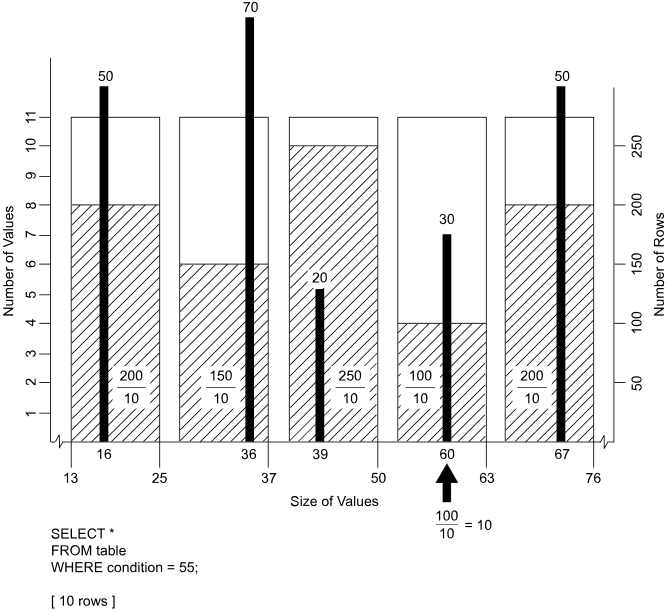• It is an equality.
• The equality ranges over a single interval.
• The most frequent value in the interval does not qualify its rows for inclusion in the response set.

The Optimizer must estimate the cardinality of the response set for this example because unlike the previous example, there are no exact statistics to describe it. The heuristic used to estimate the response set cardinality is to divide the number of rows in the interval not having the most frequent value by the number of values in the interval not equal to the most frequent value.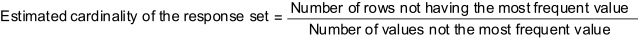If the statistics are current, then there are 100 rows in this interval that do not have the value 30 for the column specified by condition, and there are 10 values that are not equal to the most frequent value in the interval. The Optimizer divides the number of rows in the interval that do not have the value 30 by the number of values in the interval not equal to the maximum value, which is 10. The cardinality of the response set is estimated to be 10 rows.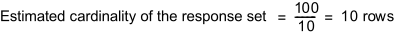This example specifies a range condition. Statistical histogram methods are a particularly powerful means for estimating the cardinalities of range query response sets.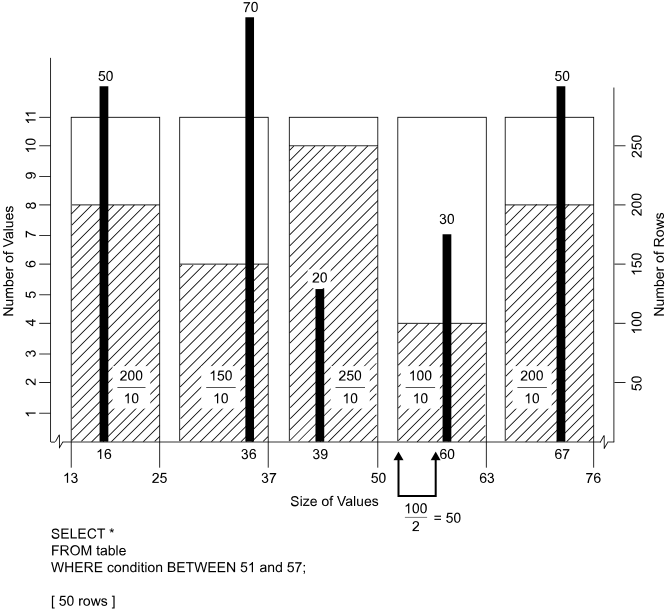The Optimizer knows that the quantity of rows having the condition column value between 51 and 57 must be found in the single interval bounded by the values 51 and 63.

The keyword BETWEEN is SQL shorthand for valuelower_limit AND ≤   upper_limit, so it signifies an inequality condition.

• It is an inequality.
• The inequality ranges over a single interval.
• The most frequent value in the interval does not qualify its rows for inclusion in the response set.

The Optimizer has to estimate the cardinality of the response set for this example because there are no exact statistics to describe it. The heuristic used to estimate the response set cardinality is to divide the number of rows not having the most frequent value in the interval in half.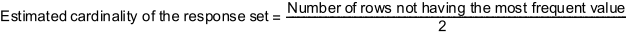Assuming current statistics, there are 100 rows in the interval that do not have the value 30 for the column specified by condition, so the Optimizer divides the number of rows not having the value 60, which is 100, in half.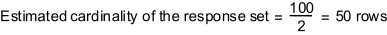The cardinality of the response set is estimated to be 50 rows.

This example is slightly more sophisticated than the previous example because it specifies a range predicate that includes the most frequently found value in the interval.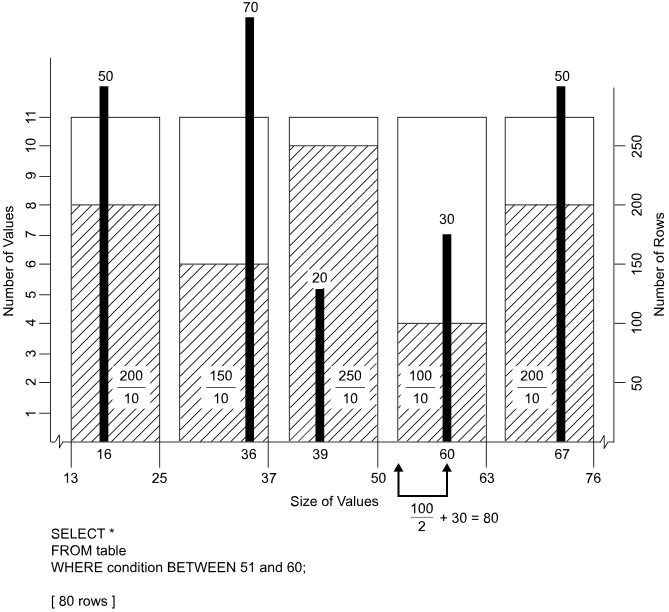The Optimizer knows that the quantity of rows having their condition column value between 51 and 60 must be found in the single interval bounded by the values 51 and 63.

• The condition is an inequality.
• The inequality ranges over a single interval.
• The most frequent value in the interval qualifies its rows for inclusion in the response set.

The Optimizer has to estimate the cardinality of the response set for this example because there are only partial exact statistics to describe it. The heuristic used to estimate the response set cardinality is to divide the number of rows not having the most frequent value in the interval in half and then add that number to the number of rows in the interval having the most frequent value.

Because this quantity is known exactly if the statistics are current, the estimated cardinality of the response set should be more accurate than in the previous example.

There are 100 rows in the interval that do not have a value 30 for the column specified by condition, so the Optimizer divides the number of rows not having the value 60, which is 100, in half and then adds the 30 rows known to exist where condition = 60.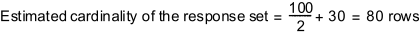This example is a slightly more complicated range query than the previous one because the response set spans 2 histogram intervals.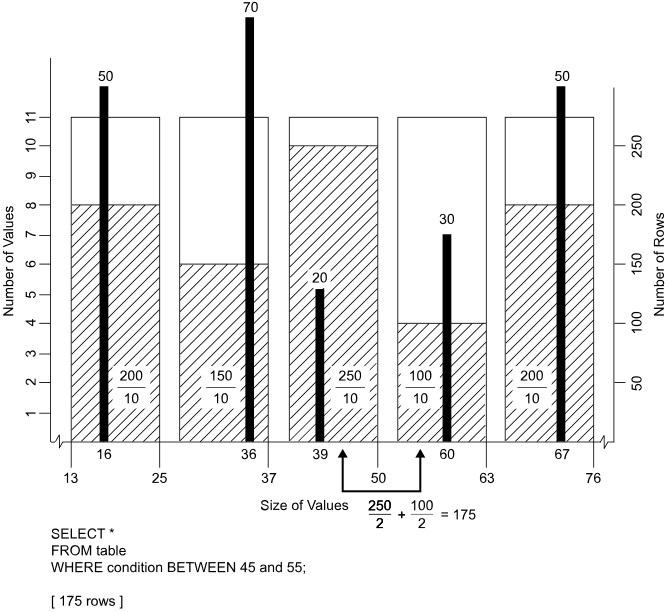The Optimizer knows that the quantity of rows having the condition column value between 45 and 55 must be found in the 2 adjacent intervals bounded by the values 38 and 63.

• It is an inequality.
• The inequality ranges over 2 intervals.
• The most frequent value in neither interval qualifies its rows for inclusion in the response set.

The Optimizer has to estimate the cardinality of the response set for this example because there are no exact statistics to describe it. The heuristic is to estimate the response set cardinalities for each interval individually by dividing the number of rows not having the most frequent value in the interval by half and then summing those 2 cardinalities.

There are 250 rows in the lower interval that do not have a value of 20 for the column specified by condition, so the Optimizer divides the number of rows not having the value 20, which is 250, in half, producing an estimate of 125 rows satisfying the condition for that interval.

There are 100 rows in the higher interval that do not have a value 30 for the column specified by condition, so the Optimizer divides the number of rows not having the value 60, which is 100, in half, producing an estimate of 50 rows satisfying the condition for that interval.

The total estimate is obtained by adding the estimates for each of the 2 intervals.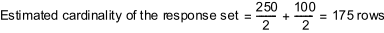The final example specifies a range query that spans three intervals and includes the most frequent value in the middle interval.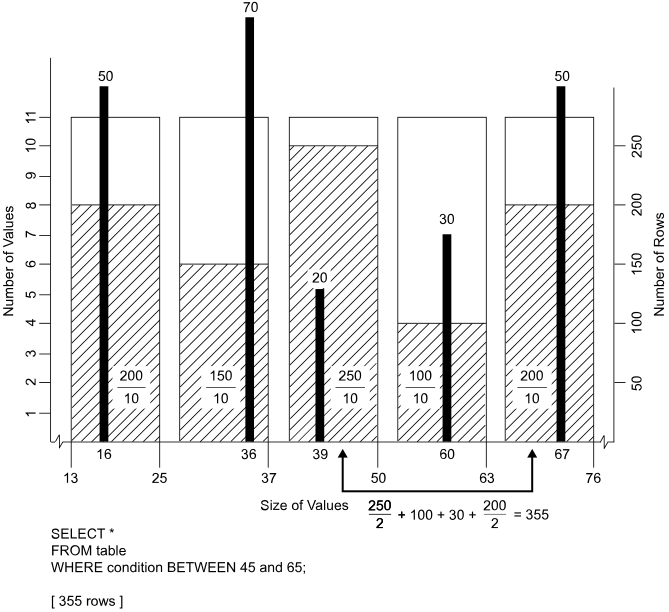The Optimizer knows that the quantity of rows having the condition column value between 45 and 50 must be found in the interval bounded by the values 38 and 50.

The Optimizer also knows that all rows in the next higher interval, which is bounded by the values 51 and 63, are included in the response set. The estimate is computed by summing the number of rows with values that are not the most frequently found within the interval with the number of rows having the most frequent value, or 100 + 30. If the statistics are current, this value is exact.

The number of rows having condition column values in the range 64 through 65 must be estimated by using half the number of values that are not the most frequently found within the interval. The estimate is half of 200, or 100 rows.

• It is an inequality.
• The inequality ranges over three intervals.
• The most frequent values in the lowest and highest intervals do not qualify their rows for inclusion in the response set.
• The most frequent value in the middle interval does qualify its rows for inclusion in the response set.

The Optimizer has to estimate the cardinality of the response set for this example because there are only partial exact statistics to describe it. The heuristic used to estimate the response set cardinality is as in the list that follows:

1. Estimate the cardinality of the response set returned from the first interval by dividing the number of rows not having the most frequent value in half.
2. Read the cardinality of the rows having the most frequent value from the second interval.
3. Read the cardinality of the rows not having the most frequent value from the second interval.
4. Estimate the cardinality of the response set returned from the third interval by dividing the number of rows not having the most frequent value in half.
5. Add the numbers derived in steps 1 through 4 to provide an overall estimate of the cardinality of the response set.

The total estimate is obtained by adding the estimates for each of the three intervals: 125 + 130 + 100, or 355 rows.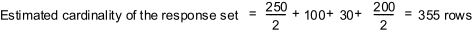Note that all of these examples are meant to show only how interval histograms are used in query optimization. They do not factor in the methods used by derived statistics and UDI counts to make cardinality estimates even more accurate.